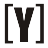# Volt-Amps (VA) to Amps (A) Calculator

To calculate VA to Amps, select phase (single or three phase) first, insert value of volt-amps and line to line volts then press Calculate Button to get the result of Amps (VA). Swap to Amps to Volt-Amps calculator.

 Select phase #: Single phase Three phase Enter volt-amps: VA Enter line to line volts: V Result in amps: A

## How to Calculate volt-amps (VA) to Amps (A)

Single phase VA to amps Formula
`I (A) = S (VA) / V (V)`

I in amps equal to the apparent power S in volt-amps, divided by the voltage V in volts.

3 phase VA to amps Formula
`I (A) = S (VA) / (√3 × VL-L (V) ) = S (VA) / (3 × VL-N (V) )`

I in amps equal to apparent power S in volt-amps, divided by the square root of 3 times the line to line voltage VL-L in volts.YoosFuhl.com Would you like to receive notifications on latest updates? No Yes